Geodesics, Exponential Map

Cosider the hyperbolic metric on R×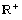defined for r>0 asThe parameter r does not change the geodesics on R×qualitatively, for r does not appear in the geodesic equations below. Although the expressions for the geodesics are simple, Mathematica has trouble solving the differential equations. Maybe in the next version...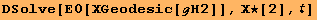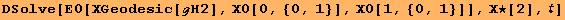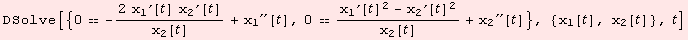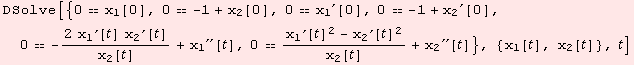For illustration purposes numerial approximation is good enough. We set r=1, but only for normalization of the initial vector.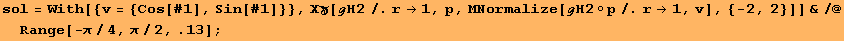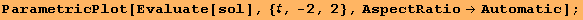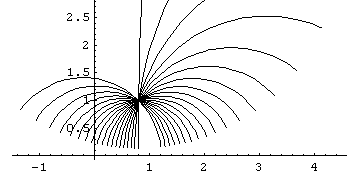We visualize the function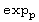: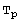M→M for p∈M for a particular point p. The lines that appear correspond to the canonical coordinate lines ofM.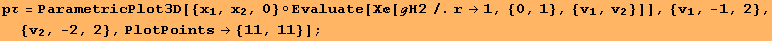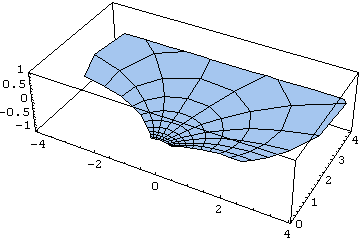Another example: The following space (M,g) is geodesically complete. However, between two points there might not be a connecting geodesic. M=(,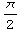R equipped with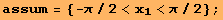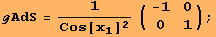Note that the metric does not depend on the coordinate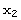.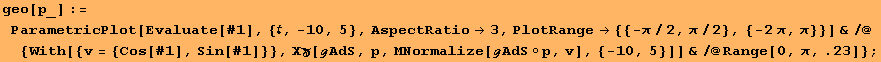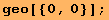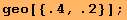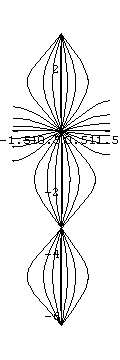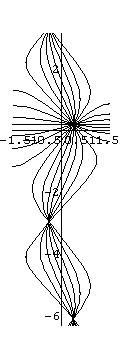The space is heavily curved near the "boundary"?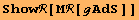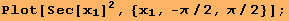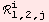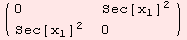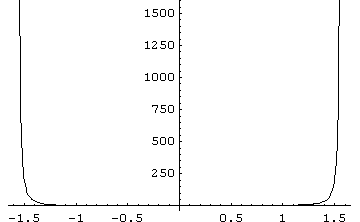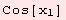Not really. The sectional curvature is constant =1 for non-degenerated planes.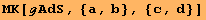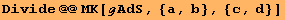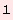Finally, the image of the exponential mapfor p=(.4,.2) with coordinate lines.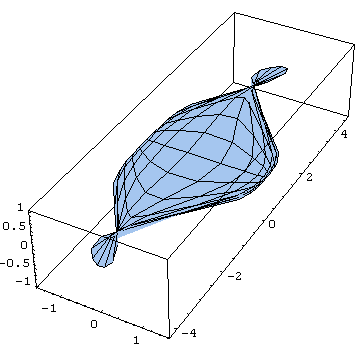Next, we consider M=<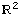,g> with a special Lorentzian metric g. The semi-Riemannian manifold M is not complete, i.e. not all geodesics are defined on the entire real line.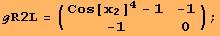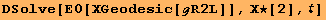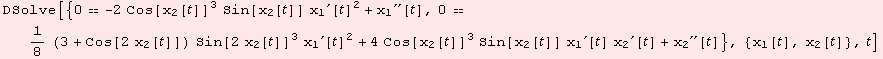We know, that γ(t)={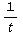-t,ArcTan[t]} is a solution. Although, lets check: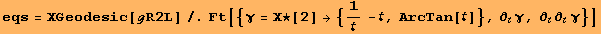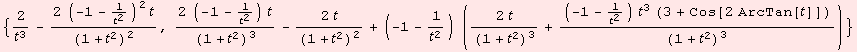Everybody can tell what happens for t→0.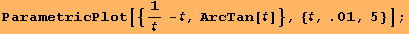Our code does not compensate for this incident.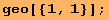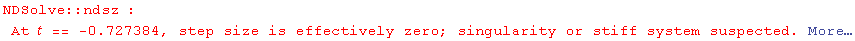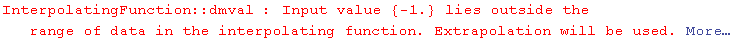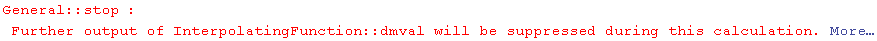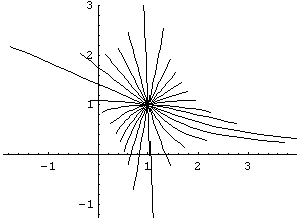Created by Mathematica  (December 22, 2006)